## Introduction to Inverse Functions

To find the inverse function, switch the $x$ and $y$ values, and then solve for $y$.

### Learning Objectives

Calculate the formula of an function’s inverse by switching $x$ and $y$ and then solving for $y$.

### Key Takeaways

#### Key Points

• An inverse function reverses the inputs and outputs.
• To find the inverse formula of a function, write it in the form of $y$ and $x$, switch $y$ and $x$, and then solve for $y$.
• Some functions have no inverse function, as a function cannot have multiple outputs.

#### Key Terms

• inverse function: A function that does exactly the opposite of another

### Definition of Inverse Function

An inverse function, which is notated $f^{-1}(x)$, is defined as the inverse function of $f(x)$ if it consistently reverses the $f(x)$ process. That is, if $f(x)$ turns $a$ into $b$, then $f^{-1}(x)$ must turn $b$ into $a$. More concisely and formally, $f^{-1}(x)$ is the inverse function of $f(x)$ if:

$f({f}^{-1}(x))=x$

Below is a mapping of function $f(x)$  and its inverse function, $f^{-1}(x)$.  Notice that the ordered pairs are reversed from the original function to its inverse.  Because $f(x)$ maps $a$ to $3$, the inverse $f^{-1}(x)$ maps $3$ back to $a$.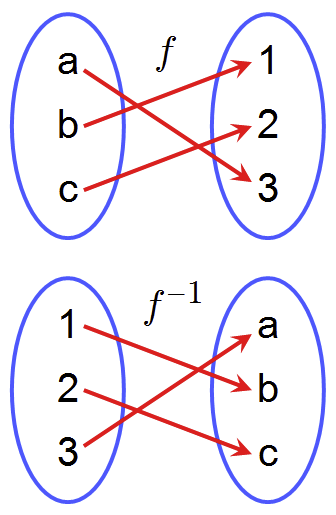Inverse functions: mapping representation: An inverse function reverses the inputs and outputs.

Thus the graph of $f^{-1}(x)$ can be obtained from the graph of $f(x)$ by switching the positions of the $x$ and $y$-axes. This is equivalent to reflecting the graph across the line $y=x$, an increasing diagonal line through the origin.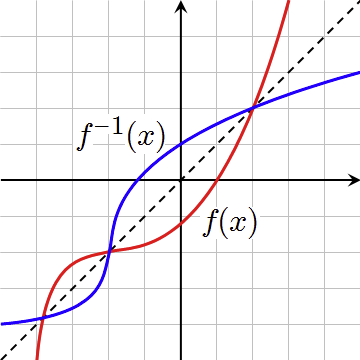Inverse functions: graphic representation: The function graph (red) and its inverse function graph (blue) are reflections of each other about the line $y=x$ (dotted black line). Notice that any ordered pair on the red curve has its reversed ordered pair on the blue line. For example, $(0,1)$ on the red (function) curve is reflected over the line $y=x$ and becomes $(1,0)$ on the blue (inverse function) curve. Where one curve is on the line $y=x$, the curves intersect, as a reflection over the line leaves the point unchanged.

### Write the Inverse Function

In general, given a function, how do you find its inverse function? Remember that an inverse function reverses the inputs and outputs.  So to find the inverse function, switch the $x$ and $y$ values of a given function, and then solve for $y$.

### Example 1

Find the inverse of: $f(x)=x^2$

a.: Write the function as:  $y=x^2$

b.: Switch the $x$ and $y$ variables: $x=y^2$

c.: Solve for $y$:

\begin {align} x&=y^2 \\ \pm\sqrt{x}&=y \end {align}

Since the function $f(x)=x^2$ has multiple outputs, its inverse is not a function. Notice the graphs in the picture below.  Even though the blue curve is a function (passes the vertical line test), its inverse would not be. The red curve for the function $f(x)=\sqrt{x}$ is not the full inverse of the function $f(x)=x^2$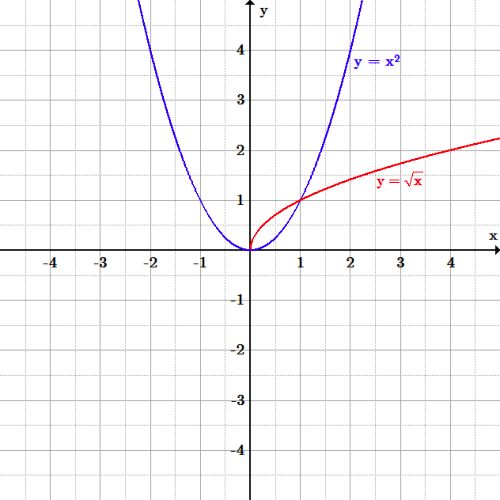The inverse is not a function: A function’s inverse may not always be a function.  The function (blue) $f(x)=x^2$, includes the points $(-1,1)$ and $(1,1)$.  Therefore, the inverse would include the points: $(1,-1)$ and $(1,1)$ which the input value repeats, and therefore is not a function. For $f(x)=\sqrt{x}$ to be a function, it must be defined as positive.

### Example 2

Find the inverse function of: $f(x)=2^x$

As soon as the problem includes an exponential function, we know that the logarithm reverses exponentiation.  The complex logarithm is the inverse function of the exponential function applied to complex numbers.  Let’s see what happens when we switch the input and output values and solve for $y$.

a.: Write the function as: $y = {2}^{x}$

b.: Switch the $x$ and $y$ variables: $x = {2}^{y}$

c.: Solve for $y$:

\begin {align} {log}_{2}x &= {log}_{2}{2}^{y} \\{log}_{2}x &= y{log}_{2}{2} \\{log}_{2}x &= y \\{f}^{1}(x) &= {log}_{2}(x) \end {align}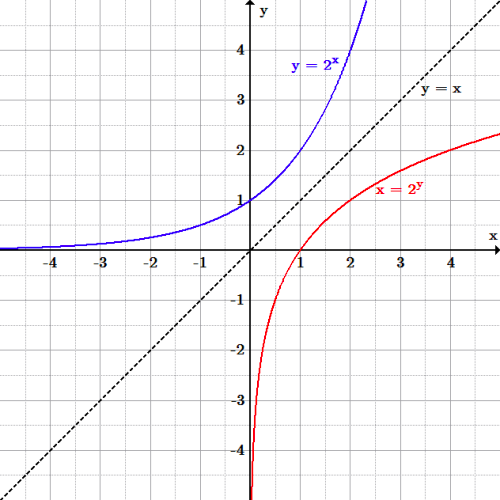Exponential and logarithm functions: The graphs of $y=2^x$ (blue) and $x=2^y$ (red) are inverses of one another. The black line represents the line of reflection, in which is $y=x$.

Test to make sure this solution fills the definition of an inverse function.

1. Pick a number, and plug it into the original function. $2\rightarrow f(x)\rightarrow 4$.
2. See if the inverse function reverses this process. $4\rightarrow f^{-1}(x)\rightarrow 2$. ✓

## Composition of Functions and Decomposing a Function

Functional composition allows for the application of one function to another; this step can be undone by using functional decomposition.

### Learning Objectives

Practice functional composition by applying the rules of one function to the results of another function

### Key Takeaways

#### Key Points

• Functional composition applies one function to the results of another.
• Functional decomposition resolves a functional relationship into its constituent parts so that the original function can be reconstructed from those parts by functional composition.
• Decomposition of a function into non-interacting components generally permits more economical representations of the function.
• The process of combining functions so that the output of one function becomes the input of another is known as a composition of functions. The resulting function is known as a composite function. We represent this combination by the following notation: $(f∘g (x)=f(g(x))$
• The domain of the composite function $(f∘g)$ is all $x$ such that $x$ is in the domain of $g$ and $g(x)$ is in the domain of $f$.

#### Key Terms

• codomain: The target space into which a function maps elements of its domain. It always contains the range of the function, but can be larger than the range if the function is not subjective.
• domain: The set of all points over which a function is defined.

### Function Composition

The process of combining functions so that the output of one function becomes the input of another is known as a composition of functions. The resulting function is known as a composite function. We represent this combination by the following notation:

$(f∘g)(x)=f(g(x))$

We read the left-hand side as “$f$“ composed with $g$ at $x$, and the right-hand side as  “$f$ of $g$ of $x$.”  The two sides of the equation have the same mathematical meaning and are equal.  The open circle symbol, $∘$, is called the composition operator. Composition is a binary operation that takes two functions and forms a new function, much as addition or multiplication takes two numbers and gives a new number.

### Function Composition and Evaluation

It is important to understand the order of operations in evaluating a composite function. We follow the usual convention with parentheses by starting with the innermost parentheses first, and then working to the outside.

In general, $(f∘g)$ and $(g∘f)$ are different functions. In other words, in many cases $f(g(x))\ne g(f(x))$ for all $x$.

Note that the range of the inside function (the first function to be evaluated) needs to be within the domain of the outside function. Less formally, the composition has to make sense in terms of inputs and outputs.

### Evaluating Composite Functions Using Input Values

When evaluating a composite function where we have either created or been given formulas, the rule of working from the inside out remains the same. The input value to the outer function will be the output of the inner function, which may be a numerical value, a variable name, or a more complicated expression.

### Example 1

If $f(x)=-2x$ and $g(x)=x^2-1$, evaluate $f(g(3))$ and $g(f(3))$.

To evaluate $f(g(3))$, first substitute, or input the value of $3$ into $g(x)$ and find the output.  Then substitute that value into the $f(x)$ function, and simplify:

$g(3)=(3)^2-1=9-1=8$

$f(8)=-2(8)=-16$

Therefore, $f(g(3))=-16$

To evaluate $g(f(3))$, find $f(3)$ and then use that output value as the input value into the $g(x)$ function:

$f(3)=-2(3)=-6$

$g(-6)=(-6)^2-1=36-1=35$

Therefore, $g(f(3))=35$

### Evaluating Composite Functions Using a Formula

While we can compose the functions for each individual input value, it is sometimes helpful to find a single formula that will calculate the result of a composition $f(g(x))$ or $g(f(x))$. To do this, we will extend our idea of function evaluation.

In the next example we are given a formula for two composite functions and asked to evaluate the function.  Evaluate the inside function using the input value or variable provided.  Use the resulting output as the input to the outside function.

### Example 2

If $f(x) =-2x$ and $g(x)=x^2-1$, evaluate $f(g(x))$ and $g(f(x))$.

First substitute, or input the function $g(x)$, $x^2-1$ into the $f(x)$ function, and then simplify:

$f(g(x))=-2(x^2-1)$

$f(g(x))=-2x^2+2$

For $g(f(x))$, input the $f(x)$ function, $-2x$ into the $g(x)$ function, and then simplify:

$g(f(x))=(-2x)^2-1$

$g(f(x))=4x^2-1$

### Functional Decomposition

Functional decomposition broadly refers to the process of resolving a functional relationship into its constituent parts in such a way that the original function can be reconstructed (i.e., recomposed) from those parts by function composition. In general, this process of decomposition is undertaken either for the purpose of gaining insight into the identity of the constituent components (which may reflect individual physical processes of interest), or for the purpose of obtaining a compressed representation of the global function; a task which is feasible only when the constituent processes possess a certain level of modularity (i.e., independence or non-interaction).

In general, functional decompositions are worthwhile when there is a certain “sparseness” in the dependency structure; i.e. when constituent functions are found to depend on approximately disjointed sets of variables. Also, decomposition of a function into non-interacting components generally permits more economical representations of the function.

## Restricting Domains to Find Inverses

Domain restriction is important for inverse functions of exponents and logarithms because sometimes we need to find an unique inverse.

### Learning Objectives

Determine inverses of functions by restricting their domains

### Key Takeaways

#### Key Points

• $f^{-1}(x)$ is defined as the inverse function of $f(x)$ if it consistently reverses the $f(x)$process.
• Informally, a restriction of a function $f$ is the result of trimming its domain.
• $f(x)={x}^{2}$, without any domain restriction, does not have an inverse function, as it fails the horizontal line test.

#### Key Terms

• domain: The set of points over which a function is defined.

### Inverse Functions

$f^{-1}(x)$ is defined as the inverse function of $f(x)$ if it consistently reverses the $f(x)$ process. That is, if $f(x)$ turns $a$ into $b$, then $f^{-1}x$ must turn $b$ into $a$. More concisely and formally, $f^{-1}x$ is the inverse function of $f(x)$ if $f({f}^{-1}(x))=x$.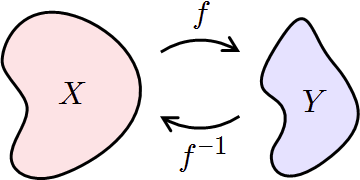Inverse functions’ domain and range: If $f$ maps $X$ to $Y$, then $f^{-1}$ maps $Y$ back to $X$.

### Domain Restrictions: Parabola

Informally, a restriction of a function is the result of trimming its domain. Remember that:

If $f$ maps $X$ to $Y$, then $f^{-1}$ maps $Y$ back to $X$.  This is not true of the function $f(x)=x^2$.

Without any domain restriction, $f(x)=x^2$ does not have an inverse function as it fails the horizontal line test. But if we restrict the domain to be $x > 0$ then we find that it passes the horizontal line test and therefore has an inverse function.  Below is the graph of the parabola and its “inverse.”  Notice that the parabola does not have a “true” inverse because the original function fails the horizontal line test and must have a restricted domain to have an inverse.Failure of horizontal line test: Graph of a parabola with the equation $y=x^2$, the U-Shaped curve opening up. This function fails the horizontal line test, and therefore does not have an inverse. The inverse equation, $y=\sqrt{x}$ (other graph) only includes the positive input values of the parabola’s domain. However, if we restrict the domain to be $x>0$, then we find that it passes the horizontal line test and will match the inverse function.

### Domain Restriction: Exponential and Logarithmic Functions

Domain restriction is important for inverse functions of exponents and logarithms because sometimes we need to find an unique inverse.  The inverse of an exponential function is a logarithmic function, and the inverse of a logarithmic function is an exponential function.

### Example 1

Is  $x=0$ in the domain of the function $f(x)=log(x)$?  If so, what is the value of the function when $x=0$?  Verify the result.

No, the function has no defined value for $x=0$. To verify, suppose $x=0$ is in the domain of the function $f(x)=log(x)$. Then there is some number $n$ such that $n=log(0)$. Rewriting as an exponential equation gives: $10n=0$, which is impossible since no such real number $n$ exists. Therefore, $x=0$ is not in the domain of the function $f(x)=log(x)$.

## Inverses of Composite Functions

A composite function represents, in one function, the results of an entire chain of dependent functions.

### Learning Objectives

Solve for the inverse of a composite function

### Key Takeaways

#### Key Points

• The composition of functions is always associative. That is, if $f$, $g$, and $h$ are three functions with suitably chosen domains and co-domains, then $f ∘ (g ∘ h) = (f ∘ g) ∘ h$, where the parentheses serve to indicate that composition is to be performed first for the parenthesized functions.
• Functions can be inverted and then composed, giving the notation of: $(f' \circ g' ) (x)$.
• Functions can be composed and then inverted, yielding the following notation: ${(f\circ g)}'(x)$.

#### Key Terms

• composite function: A function of one or more independent variables, at least one of which is itself a function of one or more other independent variables; a function of a function

### Composition and Composite Functions

In mathematics, function composition is the application of one function to the results of another.Composition of functions: $g \circ f$, the composition of $f$ and $g$. For example, $(g\circ f)(c) = \#$.

The functions $g$ and $f$ are said to commute with each other if $g ∘ f = f ∘ g$. In general, the composition of functions will not be commutative.

A composite function represents in one function the results of an entire chain of dependent functions. For example, if a school becomes larger, the supply of food in the cafeteria must become larger. This would entail ordering more sandwiches, which means ordering more ingredients, drinks, plates, etc. The entire chain of dependent functions are the ingredients, drinks, plates, etc., and the one composite function would be putting the entire chain together in order to calculate a larger population at the school.

### Inverses and Composition

Let’s go through the relationship between inverses and composition in this example. let’s take two functions, compose and invert them.

Find: $(f∘g)'(x)(f\circ g)' (x)$

\begin {align}f(x)&={x}^{2} \\g(x)&=x+1 \end {align}

First, compose them by applying the function $f$ to the result of applying function $g$:

\begin {align}(f\circ g)(x)&=f(x+1)=(x+1)^2 \\y&=(x+1)^2 \\\sqrt {y}&=x+1 \\\sqrt{y}-1&=x \end {align}

Then, invert it by switching the $x$ and $y$ variables:

\begin {align} \sqrt{x}-1&=y \\\sqrt{x}-1&=(f\circ g)' (x) \end {align}